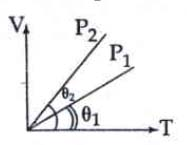#### In the given (V - T) diagram, what is the relation between pressures P1 and P2 ?Option 1) Cannot be predicted Option 2) P2 = P1 Option 3) P2 > P1 Option 4) P2 < P1As we learnt in

Equation of state -

The relation between the  Thermodynamics variable (P, V, T) of the system is called equation of state.

- whereinFor an ideal gasorFor V-T graph slopeFrom figure ,ororCorrect option is 3.

Option 1)

Cannot be predicted

Incorrect

Option 2)

P2 = P1

Incorrect

Option 3)

P> P1

Correct

Option 4)

P< P1

Incorrect